← Machine Learning Projects

# Machine Learning Engineer Nanodegree¶

## Project: Predicting Boston Housing Prices¶

Welcome to the first project of the Machine Learning Engineer Nanodegree! In this notebook, some template code has already been provided for you, and you will need to implement additional functionality to successfully complete this project. You will not need to modify the included code beyond what is requested. Sections that begin with 'Implementation' in the header indicate that the following block of code will require additional functionality which you must provide. Instructions will be provided for each section and the specifics of the implementation are marked in the code block with a 'TODO' statement. Please be sure to read the instructions carefully!

Note: Code and Markdown cells can be executed using the Shift + Enter keyboard shortcut. In addition, Markdown cells can be edited by typically double-clicking the cell to enter edit mode.

## Getting Started¶

In this project, you will evaluate the performance and predictive power of a model that has been trained and tested on data collected from homes in suburbs of Boston, Massachusetts. A model trained on this data that is seen as a good fit could then be used to make certain predictions about a home — in particular, its monetary value. This model would prove to be invaluable for someone like a real estate agent who could make use of such information on a daily basis.

The dataset for this project originates from the UCI Machine Learning Repository. The Boston housing data was collected in 1978 and each of the 506 entries represent aggregated data about 14 features for homes from various suburbs in Boston, Massachusetts. For the purposes of this project, the following preprocessing steps have been made to the dataset:

• 16 data points have an 'MEDV' value of 50.0. These data points likely contain missing or censored values and have been removed.
• 1 data point has an 'RM' value of 8.78. This data point can be considered an outlier and has been removed.
• The features 'RM', 'LSTAT', 'PTRATIO', and 'MEDV' are essential. The remaining non-relevant features have been excluded.
• The feature 'MEDV' has been multiplicatively scaled to account for 35 years of market inflation.

Run the code cell below to load the Boston housing dataset, along with a few of the necessary Python libraries required for this project. You will know the dataset loaded successfully if the size of the dataset is reported.

In :
# Import libraries necessary for this project
import numpy as np
import pandas as pd
from sklearn.cross_validation import ShuffleSplit

# Import supplementary visualizations code visuals.py
import visuals as vs

# Pretty display for notebooks
%matplotlib inline

# Load the Boston housing dataset
prices = data['MEDV']
features = data.drop('MEDV', axis = 1)

# Success
print "Boston housing dataset has {} data points with {} variables each.".format(*data.shape)

Boston housing dataset has 489 data points with 4 variables each.


## Data Exploration¶

In this first section of this project, you will make a cursory investigation about the Boston housing data and provide your observations. Familiarizing yourself with the data through an explorative process is a fundamental practice to help you better understand and justify your results.

Since the main goal of this project is to construct a working model which has the capability of predicting the value of houses, we will need to separate the dataset into features and the target variable. The features, 'RM', 'LSTAT', and 'PTRATIO', give us quantitative information about each data point. The target variable, 'MEDV', will be the variable we seek to predict. These are stored in features and prices, respectively.

### Implementation: Calculate Statistics¶

For your very first coding implementation, you will calculate descriptive statistics about the Boston housing prices. Since numpy has already been imported for you, use this library to perform the necessary calculations. These statistics will be extremely important later on to analyze various prediction results from the constructed model.

In the code cell below, you will need to implement the following:

• Calculate the minimum, maximum, mean, median, and standard deviation of 'MEDV', which is stored in prices.
• Store each calculation in their respective variable.
In :
import matplotlib.pyplot as plt
import numpy as np

for col in features.columns:

fig, ax = plt.subplots()
fit = np.polyfit(features [col], prices, deg=1) # We use a linear fit to compute the trendline
ax.scatter(features [col],  prices)
plt.plot(features [col], prices, 'o', color='black')
ax.plot(features[col], fit * features[col] + fit, color='blue', linewidth=3) # This plots a trendline with the regression parameters computed earlier. We should plot this after the dots or it will be covered by the dots themselves
plt.title('PRICES vs  '+ str(col)) # title here
plt.xlabel(col) # label here
plt.ylabel('PRICES') # label here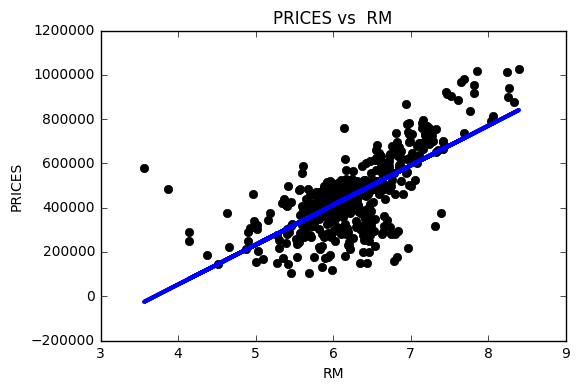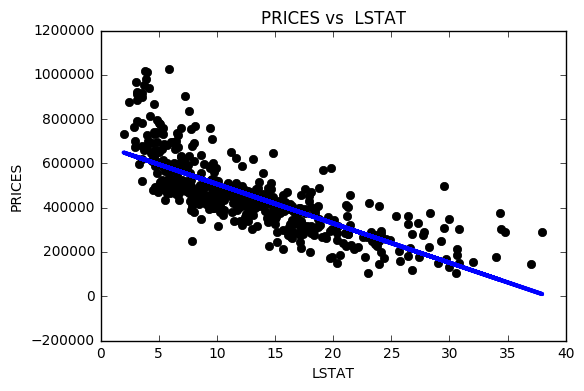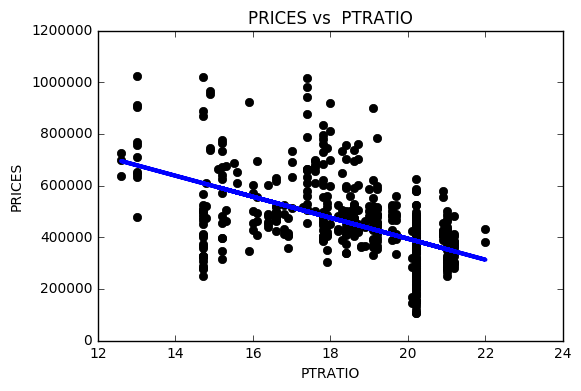In :
# TODO: Minimum price of the data
minimum_price = np.min(prices)

# TODO: Maximum price of the data
maximum_price = np.max(prices)

# TODO: Mean price of the data
mean_price = np.mean(prices)

# TODO: Median price of the data
median_price = np.median(prices)

# TODO: Standard deviation of prices of the data
std_price = np.std(prices)

# Show the calculated statistics
print "Statistics for Boston housing dataset:\n"
print "Minimum price: ${:,.2f}".format(minimum_price) print "Maximum price:${:,.2f}".format(maximum_price)
print "Mean price: ${:,.2f}".format(mean_price) print "Median price${:,.2f}".format(median_price)
print "Standard deviation of prices: ${:,.2f}".format(std_price)  Statistics for Boston housing dataset: Minimum price:$105,000.00
Maximum price: $1,024,800.00 Mean price:$454,342.94
Median price $438,900.00 Standard deviation of prices:$165,171.13


### Question 1 - Feature Observation¶

As a reminder, we are using three features from the Boston housing dataset: 'RM', 'LSTAT', and 'PTRATIO'. For each data point (neighborhood):

• 'RM' is the average number of rooms among homes in the neighborhood.
• 'LSTAT' is the percentage of homeowners in the neighborhood considered "lower class" (working poor).
• 'PTRATIO' is the ratio of students to teachers in primary and secondary schools in the neighborhood.

Using your intuition, for each of the three features above, do you think that an increase in the value of that feature would lead to an increase in the value of 'MEDV' or a decrease in the value of 'MEDV'? Justify your answer for each.
Hint: Would you expect a home that has an 'RM' value of 6 be worth more or less than a home that has an 'RM' value of 7?

1. I think an increase in the value of 'RM' would increase the value of 'MEDV', as houses that typically have a larger number of bedrooms are more expensive, because they typically occupy a higher square footage than those with a lesser number of bedrooms.

2. I think an increase in the value of 'LSTAT' would lead to a decrease in the value of 'MEDV', as a higher percentage of homeowners in the neighborhood being considered working poor would mean that the houses are more affordable (cheaper).

3. I think an increase in the ratio of students to teachers in primary and secondary schools in the neighborhood would indicate that the area is more densely populated, which is often associated with less affulent neighborhoods. Private schools typically tend to have a lower student to teacher ratio than public schools (especially public schools in inner city areas), and are more likely to be found in more affluent neighborhoods. So an increase in the value of 'PTRATIO' would lead to a decrease in the value of 'MEDV'.

## Developing a Model¶

In this second section of the project, you will develop the tools and techniques necessary for a model to make a prediction. Being able to make accurate evaluations of each model's performance through the use of these tools and techniques helps to greatly reinforce the confidence in your predictions.

### Implementation: Define a Performance Metric¶

It is difficult to measure the quality of a given model without quantifying its performance over training and testing. This is typically done using some type of performance metric, whether it is through calculating some type of error, the goodness of fit, or some other useful measurement. For this project, you will be calculating the coefficient of determination, R2, to quantify your model's performance. The coefficient of determination for a model is a useful statistic in regression analysis, as it often describes how "good" that model is at making predictions.

The values for R2 range from 0 to 1, which captures the percentage of squared correlation between the predicted and actual values of the target variable. A model with an R2 of 0 is no better than a model that always predicts the mean of the target variable, whereas a model with an R2 of 1 perfectly predicts the target variable. Any value between 0 and 1 indicates what percentage of the target variable, using this model, can be explained by the features. A model can be given a negative R2 as well, which indicates that the model is arbitrarily worse than one that always predicts the mean of the target variable.

For the performance_metric function in the code cell below, you will need to implement the following:

• Use r2_score from sklearn.metrics to perform a performance calculation between y_true and y_predict.
• Assign the performance score to the score variable.
In :
# TODO: Import 'r2_score'

from sklearn.metrics import r2_score

def performance_metric(y_true, y_predict):
""" Calculates and returns the performance score between
true and predicted values based on the metric chosen. """

# TODO: Calculate the performance score between 'y_true' and 'y_predict'
score = r2_score(y_true, y_predict)

# Return the score
return score


### Question 2 - Goodness of Fit¶

Assume that a dataset contains five data points and a model made the following predictions for the target variable:

True Value Prediction
3.0 2.5
-0.5 0.0
2.0 2.1
7.0 7.8
4.2 5.3

Would you consider this model to have successfully captured the variation of the target variable? Why or why not?

Run the code cell below to use the performance_metric function and calculate this model's coefficient of determination.

In :
%matplotlib inline

true, pred = [3, -0.5, 2, 7, 4.2], [2.5, 0.0, 2.1, 7.8, 5.3]
#Plot true values
true_handle = plt.scatter(true, true, alpha=0.6, color='blue', label='true')

#Reference line
fit = np.poly1d(np.polyfit(true, true, 1))
lims = np.linspace(min(true) - 1, max(true) + 1)
plt.plot(lims, fit(lims), alpha=0.3, color='black')

#Plot predicted values
pred_handle = plt.scatter(true, pred, alpha=0.6, color='red', label='predicted')

#Legend and show
plt.legend(handles=[true_handle, pred_handle], loc='upper left')
plt.show()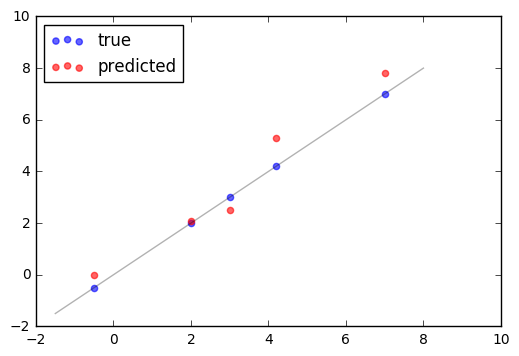In :
# Calculate the performance of this model
score = performance_metric([3, -0.5, 2, 7, 4.2], [2.5, 0.0, 2.1, 7.8, 5.3])
print "Model has a coefficient of determination, R^2, of {:.3f}.".format(score)

Model has a coefficient of determination, R^2, of 0.923.


This model has successfully captured the variation of the target variable, as an R^2 score of 0.923 is very high. This indicates that 92.3% of the target variable can be explained by the model's features.

### Implementation: Shuffle and Split Data¶

Your next implementation requires that you take the Boston housing dataset and split the data into training and testing subsets. Typically, the data is also shuffled into a random order when creating the training and testing subsets to remove any bias in the ordering of the dataset.

For the code cell below, you will need to implement the following:

• Use train_test_split from sklearn.cross_validation to shuffle and split the features and prices data into training and testing sets.
• Split the data into 80% training and 20% testing.
• Set the random_state for train_test_split to a value of your choice. This ensures results are consistent.
• Assign the train and testing splits to X_train, X_test, y_train, and y_test.
In :
# TODO: Import 'train_test_split'

from sklearn.cross_validation import train_test_split

X = prices
y = features

# TODO: Shuffle and split the data into training and testing subsets
X_train, X_test, y_train, y_test = train_test_split(X, y, test_size = 0.2, random_state = 0)

# Success
print "Training and testing split was successful."

Training and testing split was successful.


### Question 3 - Training and Testing¶

What is the benefit to splitting a dataset into some ratio of training and testing subsets for a learning algorithm?
Hint: What could go wrong with not having a way to test your model?

One benefit of splitting a dataset into some ratio of training and testing subsets is that it prevents 'overfitting', a problem where the model has become too finely tuned to the data its been given so that it is unable to create accurate predictions on data it hasn't been trained on. It allows us to test our model on an independent dataset so we can estimate its performance. Not having a way to test your model would prevent you from being able to assess the quality of your model.

## Analyzing Model Performance¶

In this third section of the project, you'll take a look at several models' learning and testing performances on various subsets of training data. Additionally, you'll investigate one particular algorithm with an increasing 'max_depth' parameter on the full training set to observe how model complexity affects performance. Graphing your model's performance based on varying criteria can be beneficial in the analysis process, such as visualizing behavior that may not have been apparent from the results alone.

### Learning Curves¶

The following code cell produces four graphs for a decision tree model with different maximum depths. Each graph visualizes the learning curves of the model for both training and testing as the size of the training set is increased. Note that the shaded region of a learning curve denotes the uncertainty of that curve (measured as the standard deviation). The model is scored on both the training and testing sets using R2, the coefficient of determination.

Run the code cell below and use these graphs to answer the following question.

In :
# Produce learning curves for varying training set sizes and maximum depths
vs.ModelLearning(features, prices)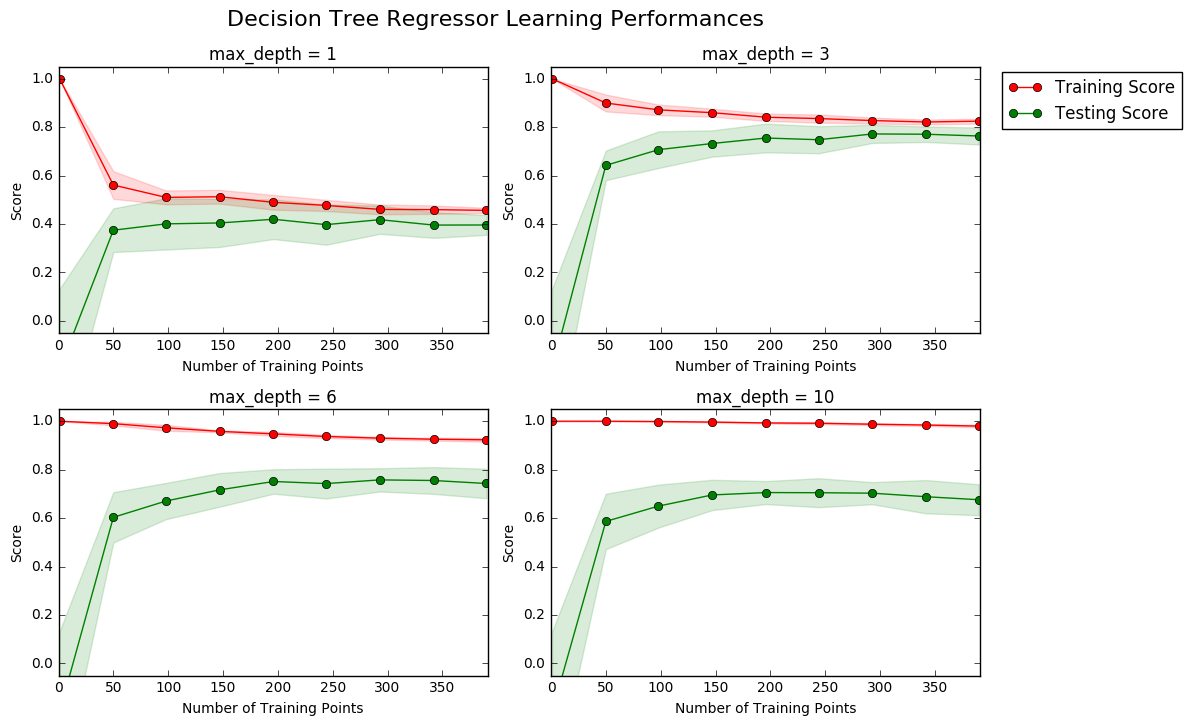### Question 4 - Learning the Data¶

Choose one of the graphs above and state the maximum depth for the model. What happens to the score of the training curve as more training points are added? What about the testing curve? Would having more training points benefit the model?
Hint: Are the learning curves converging to particular scores?

I decided to look at the graph with a maximum depth of 3, as it was the model with the highest testing score. The score of the training curve decreases as more training points are added, though it appears to level off after around 300 training points have been used. The score of the testing curve generally increases as more training points are added, though it also appears to level off after a number of 300 training points have been used (and appears to decrease slightly after this point). Having greater than 300 training points doesn't appear to benefit the model.

### Complexity Curves¶

The following code cell produces a graph for a decision tree model that has been trained and validated on the training data using different maximum depths. The graph produces two complexity curves — one for training and one for validation. Similar to the learning curves, the shaded regions of both the complexity curves denote the uncertainty in those curves, and the model is scored on both the training and validation sets using the performance_metric function.

Run the code cell below and use this graph to answer the following two questions.

In :
vs.ModelComplexity(y_train, X_train)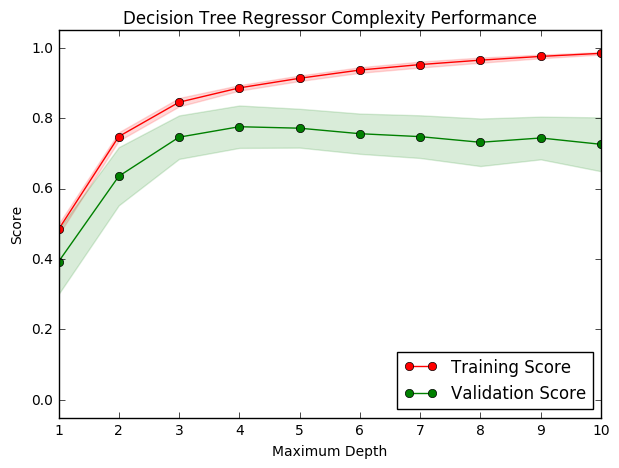### Question 5 - Bias-Variance Tradeoff¶

When the model is trained with a maximum depth of 1, does the model suffer from high bias or from high variance? How about when the model is trained with a maximum depth of 10? What visual cues in the graph justify your conclusions?
Hint: How do you know when a model is suffering from high bias or high variance?

When the model is trained with a maximum depth of 1 (less complex), the model is likely to suffer more from high bias as both its training score and validation score are quite low, since the model isn't complex enough to learn the data. When the model is trained with a maximum depth of 10 (more complex), it is more likely to suffer from high variance as the difference between the training and validation score is quite large -- meaning that the model is likely to be suffering from 'overfitting', where the training score continues to improve by learning random noise in the data, while the validation score suffers due to the model losing its capacity to generalise previously unseen data.

### Question 6 - Best-Guess Optimal Model¶

Which maximum depth do you think results in a model that best generalizes to unseen data? What intuition lead you to this answer?

I think a maximum depth of 4 results in a model that best generalises to unseen data, as it yields the highest validation score (which tells us how well the model performs on unseen data).

## Evaluating Model Performance¶

In this final section of the project, you will construct a model and make a prediction on the client's feature set using an optimized model from fit_model.

What is the grid search technique and how it can be applied to optimize a learning algorithm?

The grid search technique is a way of working through different combinations of parameter tunes by cross-validating to determine which tune performs the best. It optimizes a learning algorithm by providing the best possible combination of parameters for the model, doing so by building and evaluating a model for each combination of algorithm parameters specified in a grid.

### Question 8 - Cross-Validation¶

What is the k-fold cross-validation training technique? What benefit does this technique provide for grid search when optimizing a model?
Hint: Much like the reasoning behind having a testing set, what could go wrong with using grid search without a cross-validated set?

The k-fold cross-validation training technique comprises partioning the data set into k bins of equal size, then running k learning experiments using each testing set, and averaging the test results from those k experiments. What's great about this technique is that it allows you to use all of your data for both training and testing, while there is an inherent a trade-off in normal cross-validation between maximising your training and testing data, since increasing one would lead to decreasing the other. The benefit k-fold cross-validation provides for grid search when optimising a model is that it is a hedge against overfitting, since it would allow you to grid search and cross-validate against multiple iterations of a train/test split, rather than just finding the optimal parameters for one train/test split.

### Implementation: Fitting a Model¶

Your final implementation requires that you bring everything together and train a model using the decision tree algorithm. To ensure that you are producing an optimized model, you will train the model using the grid search technique to optimize the 'max_depth' parameter for the decision tree. The 'max_depth' parameter can be thought of as how many questions the decision tree algorithm is allowed to ask about the data before making a prediction. Decision trees are part of a class of algorithms called supervised learning algorithms.

In addition, you will find your implementation is using ShuffleSplit() for an alternative form of cross-validation (see the 'cv_sets' variable). While it is not the K-Fold cross-validation technique you describe in Question 8, this type of cross-validation technique is just as useful!. The ShuffleSplit() implementation below will create 10 ('n_iter') shuffled sets, and for each shuffle, 20% ('test_size') of the data will be used as the validation set. While you're working on your implementation, think about the contrasts and similarities it has to the K-fold cross-validation technique.

For the fit_model function in the code cell below, you will need to implement the following:

• Use DecisionTreeRegressor from sklearn.tree to create a decision tree regressor object.
• Assign this object to the 'regressor' variable.
• Create a dictionary for 'max_depth' with the values from 1 to 10, and assign this to the 'params' variable.
• Use make_scorer from sklearn.metrics to create a scoring function object.
• Pass the performance_metric function as a parameter to the object.
• Assign this scoring function to the 'scoring_fnc' variable.
• Use GridSearchCV from sklearn.grid_search to create a grid search object.
• Pass the variables 'regressor', 'params', 'scoring_fnc', and 'cv_sets' as parameters to the object.
• Assign the GridSearchCV object to the 'grid' variable.
In :
# TODO: Import 'make_scorer', 'DecisionTreeRegressor', and 'GridSearchCV'

from sklearn.metrics import make_scorer
from sklearn.tree import DecisionTreeRegressor
from sklearn.grid_search import GridSearchCV

def fit_model(X, y):
""" Performs grid search over the 'max_depth' parameter for a
decision tree regressor trained on the input data [X, y]. """

# Create cross-validation sets from the training data
cv_sets = ShuffleSplit(X.shape, n_iter = 10, test_size = 0.20, random_state = 0)

# TODO: Create a decision tree regressor object
regressor = DecisionTreeRegressor()

# TODO: Create a dictionary for the parameter 'max_depth' with a range from 1 to 10
params = {'max_depth':range(1,11)}

# TODO: Transform 'performance_metric' into a scoring function using 'make_scorer'
scoring_fnc = make_scorer(performance_metric)

# TODO: Create the grid search object
grid = GridSearchCV(regressor, params, scoring_fnc, cv=cv_sets)

# Fit the grid search object to the data to compute the optimal model
grid = grid.fit(y, X)

# Return the optimal model after fitting the data
return grid.best_estimator_


### Making Predictions¶

Once a model has been trained on a given set of data, it can now be used to make predictions on new sets of input data. In the case of a decision tree regressor, the model has learned what the best questions to ask about the input data are, and can respond with a prediction for the target variable. You can use these predictions to gain information about data where the value of the target variable is unknown — such as data the model was not trained on.

### Question 9 - Optimal Model¶

What maximum depth does the optimal model have? How does this result compare to your guess in Question 6?

Run the code block below to fit the decision tree regressor to the training data and produce an optimal model.

In :
# Fit the training data to the model using grid search
reg = fit_model(X_train, y_train)

# Produce the value for 'max_depth'
print "Parameter 'max_depth' is {} for the optimal model.".format(reg.get_params()['max_depth'])

Parameter 'max_depth' is 4 for the optimal model.


The optimal model has a maximum depth of 4, which validates the (educated) guess I made in Question 6.

### Question 10 - Predicting Selling Prices¶

Imagine that you were a real estate agent in the Boston area looking to use this model to help price homes owned by your clients that they wish to sell. You have collected the following information from three of your clients:

Feature Client 1 Client 2 Client 3
Total number of rooms in home 5 rooms 4 rooms 8 rooms
Neighborhood poverty level (as %) 17% 32% 3%
Student-teacher ratio of nearby schools 15-to-1 22-to-1 12-to-1

What price would you recommend each client sell his/her home at? Do these prices seem reasonable given the values for the respective features?
Hint: Use the statistics you calculated in the Data Exploration section to help justify your response.

Run the code block below to have your optimized model make predictions for each client's home.

In :
# Produce a matrix for client data
client_data = [[5, 17, 15], # Client 1
[4, 32, 22], # Client 2
[8, 3, 12]]  # Client 3

# Show predictions
for i, price in enumerate(reg.predict(client_data)):
print "Predicted selling price for Client {}'s home: ${:,.2f}".format(i+1, price)  Predicted selling price for Client 1's home:$391,183.33
Predicted selling price for Client 2's home: $189,123.53 Predicted selling price for Client 3's home:$942,666.67

In :
clients = np.transpose(client_data)
pred = reg.predict(client_data)
for i, feat in enumerate(['RM', 'LSTAT', 'PTRATIO']):
plt.scatter(features[feat], prices, alpha=0.25, c=prices)
plt.scatter(clients[i], pred, color='black', marker='x', linewidths=2)
plt.xlabel(feat)
plt.ylabel('MEDV')
plt.show()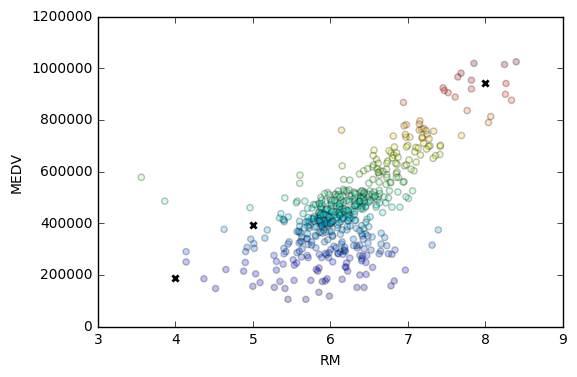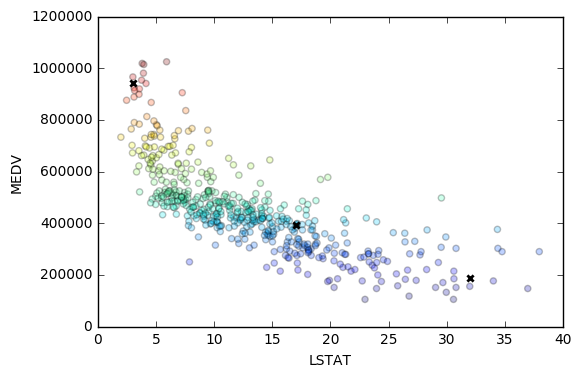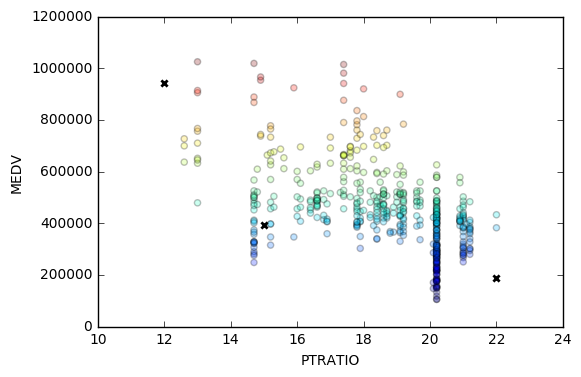To reiterate what we found in the summary statistics:

Minimum price: $105,000.00 Maximum price:$1,024,800.00

Mean price: $454,342.94 Median price$438,900.00

Standard deviation of prices: $165,171.13 Client 1: We would recommend Client 1 to sell their home for$391,183.33. This would put the house under both the median and mean price. It seems like a reasonable prediction given the somewhat high level of neighborhood poverty (17%), average number of rooms (5), and average Student-teacher ratio of nearby schools (15-to-1).

Client 2: We would recommend Client 2 to sell their home for $189,123.53. This would put the house within 2 standard deviations of both the median and mean prices. It seems like a reasonable prediction given the particularly high neighborhood poverty rate (32%), low number of rooms (4), and high Student-teacher ratio of nearby schools (22-to-1). Client 3: We would recommend Client 3 to sell their home for$942,666.67. This would put the house close to the maximum price in our data set. It seems like a reasonable prediction given the very low level of neighborhood poverty (3%), particularly high number of rooms (8), and low Student-teacher ratio of nearby schools (12-to-1).

At a glance, my intuition says that the most explanatory factor within this sample is the level of neighborhood poverty.

### Sensitivity¶

An optimal model is not necessarily a robust model. Sometimes, a model is either too complex or too simple to sufficiently generalize to new data. Sometimes, a model could use a learning algorithm that is not appropriate for the structure of the data given. Other times, the data itself could be too noisy or contain too few samples to allow a model to adequately capture the target variable — i.e., the model is underfitted. Run the code cell below to run the fit_model function ten times with different training and testing sets to see how the prediction for a specific client changes with the data it's trained on.

In :
vs.PredictTrials(prices, features, fit_model, client_data)

Trial 1: $391,183.33 Trial 2:$424,935.00
Trial 3: $415,800.00 Trial 4:$420,622.22
Trial 5: $418,377.27 Trial 6:$411,931.58
Trial 7: $399,663.16 Trial 8:$407,232.00
Trial 9: $351,577.61 Trial 10:$413,700.00

Range in prices: $73,357.39  ### Question 11 - Applicability¶ In a few sentences, discuss whether the constructed model should or should not be used in a real-world setting. Hint: Some questions to answering: • How relevant today is data that was collected from 1978? • Are the features present in the data sufficient to describe a home? • Is the model robust enough to make consistent predictions? • Would data collected in an urban city like Boston be applicable in a rural city? Answer: Data that was collected from 1978 is not relevant today due to the massive spike in house prices over the past few decades. Looking at the Case-Shiller Index for Boston alone (whose records only begin in 1987), in the decade span of 1996 to 2006 the Index increased by over 100 points. The increase in house prices during the period of 1978 to 2017 would far outpace inflation, so even if the data were adjusted for inflation, it would still fail to predict the true value of a home today. The typical determinants for current market value and future growth potential of a home are the local job market, quality of local schools, and general quality of life of the area. With these factors held constant, a significant determinant in home value is sq footage. Our features are able to capture aspects of the quality of schools (through the Student-teacher ratio), local job market and quality of life (through Neighborhood poverty level), and sq footage (through Total number of rooms in home), so it does an adequate job. However, the variables we are using are less direct measures than alternative metrics that could be used e.g. median household salary, national ranking of schools, and sq footage of the property. The fact the model has a range of \$73,357.39 for the 10 trials performed above (which equates to 17.26% of the value of the most expensive estimate, and 20.87% of the value of the least expensive estimate) means that the model may not be robust enough to make consistent predictions. That being said, the lowest value of \$351,577.61 is quite a bit lower than the rest of the predictions, which tend to hover around the \$407,000 mark, so it may be this one data point that is making the model seem less robust than it is. In fact, were the lowest point to be removed, the range would be reduced substantially to \\$33,751.67, making the range closer to below 10% of the property's value. This is still quite a high range for accurately predicting the price of the home, so I would say the model is not robust enough to make consistent predictions.

We wouldn't expect data collected in an urban city like Boston to be applicable in a rural city, because the demand for housing in urban areas is typically much greater than that for rural areas. For the same number of rooms in a home, neigborhood poverty level, and student-teacher ratio you would expect to pay a lot less in Keystone, Virginia as you would in Boston, Massachusetts. As such, if your model is used to predict houses cities outside of Boston, it could overestimate the value of the home in other areas, particularly if they are in less affluent rural communities.

Note: Once you have completed all of the code implementations and successfully answered each question above, you may finalize your work by exporting the iPython Notebook as an HTML document. You can do this by using the menu above and navigating to
File -> Download as -> HTML (.html). Include the finished document along with this notebook as your submission.